#### 期刊菜单

A New Kind of Cubic Li’s Oval
DOI: 10.12677/AAM.2022.115330, PDF, HTML, XML, 下载: 63  浏览: 78

Abstract: A definition of Li’s oval is provided, and the definitions of the oval center, and the long radius, and the short radius, and the symmetrical radius of the oval are provided. A kind of cubic equation is given and proved to be Li’s oval equation. The judgment theorems that some cubic equations are Li’s oval equation is derived, the perimeter and area of Li’s oval and the surface area and volume of rotating oval ball are deduced.

1. 引言

2. 定义

①L有一条对称轴且是闭合的；

②L上有唯一一对对称点，设为S，T到对称轴的距离最大；

③L与其对称轴有且仅有两个交点，设为P，Q，又PQ与ST交于一点设为O，且 $OP>OQ$

④L处处光滑，或者除点P，Q外处处光滑。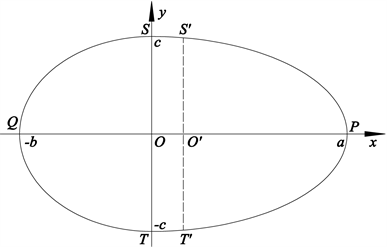Figure 1. Li’s oval and its rectangular coordinate system

3. 又一类三次李氏卵圆

$a,b,c$$>0$$b (1)

$\frac{-\left(a-b\right){x}^{3}+\left({a}^{2}-ab+{b}^{2}\right){x}^{2}}{{\left(ab\right)}^{2}}+\frac{{y}^{2}}{{c}^{2}}=1,\text{\hspace{0.17em}}x\in \left[-b,a\right]$ (2)

$y=±\frac{c}{ab}\sqrt{\left(x+b\right)\left(x-a\right)\left[\left(a-b\right)x-ab\right]},\text{\hspace{0.17em}}x\in \left[-b,a\right]$ (3)

${x}^{3}-\frac{{a}^{2}-ab+{b}^{2}}{a-b}{x}^{2}-\frac{{a}^{2}{b}^{2}}{\left(a-b\right){c}^{2}}{y}^{2}+\frac{{a}^{2}{b}^{2}}{a-b}=0,\text{\hspace{0.17em}}x\in \left[-b,a\right]$ (4)

$\left(a-b\right){c}^{2}{x}^{3}-\left({a}^{2}-ab+{b}^{2}\right){c}^{2}{x}^{2}-{a}^{2}{b}^{2}{y}^{2}+{a}^{2}{b}^{2}{c}^{2}=0,\text{\hspace{0.17em}}x\in \left[-b,a\right]$ (5)

$\frac{\left(a-b\right){y}^{3}+\left({a}^{2}-ab+{b}^{2}\right){y}^{2}}{{\left(ab\right)}^{2}}+\frac{{x}^{2}}{{c}^{2}}=1,\text{\hspace{0.17em}}y\in \left[-a,b\right]$ (6)

$x=±\frac{c}{ab}\sqrt{\left(y+a\right)\left(y-b\right)\left[\left(a-b\right)y+ab\right]},\text{\hspace{0.17em}}y\in \left[-a,b\right]$ (7)

${y}^{3}+\frac{{a}^{2}-ab+{b}^{2}}{a-b}{y}^{2}+\frac{{a}^{2}{b}^{2}}{\left(a-b\right){c}^{2}}{x}^{2}-\frac{{a}^{2}{b}^{2}}{a-b}=0,\text{\hspace{0.17em}}y\in \left[-a,b\right]$ (8)

$\left(a-b\right){c}^{2}{y}^{3}+\left({a}^{2}-ab+{b}^{2}\right){c}^{2}{y}^{2}+{a}^{2}{b}^{2}{x}^{2}-{a}^{2}{b}^{2}{c}^{2}=0,\text{\hspace{0.17em}}x\in \left[-a,b\right]$ (9)

$\left\{\begin{array}{l}x=c\mathrm{cos}t\\ \left(a-b\right){y}^{3}+\left({a}^{2}-ab+{b}^{2}\right){y}^{2}-{a}^{2}{b}^{2}{\mathrm{sin}}^{2}t=0\end{array},\text{\hspace{0.17em}}t\in \left[0,2\pi \right]$ (10)

$\left\{\begin{array}{l}y>0,\text{\hspace{0.17em}}t\in \left(0,\pi \right)\\ y<0,\text{\hspace{0.17em}}t\in \left(\pi ,2\pi \right)\\ y\left(0\right)=y\left(\pi \right)=y\left(2\pi \right)=0\end{array}$ (11)

$e=\frac{a+b}{2},\text{\hspace{0.17em}}h=\frac{a-b}{2},\text{\hspace{0.17em}}g=\frac{c\left(a+b\right)}{2\sqrt{2}ab}\sqrt{4ab-{a}^{2}-{b}^{2}}$ (12)

$\frac{\left(a-b\right){y}^{3}+\left({a}^{2}-ab+{b}^{2}\right){y}^{2}}{{\left(ab\right)}^{2}}={\mathrm{sin}}^{2}t$ (13)

$\frac{{x}^{2}}{{c}^{2}}={\mathrm{cos}}^{2}t$ (14)

(i)

${{y}^{\prime }}_{x}\left(t\right)=-\frac{2ab}{cy}\cdot \frac{\mathrm{cos}t}{h\left(y\right)}$ (15)

${{y}^{″}}_{x}\left(t\right)=\frac{2{a}^{2}{b}^{2}}{{c}^{2}{y}^{3}}\cdot \frac{w\left(t\right)}{{h}^{3}\left(y\right)}$ (16)

$h\left(y\right)=3\left(a-b\right)y+2\left({a}^{2}-ab+{b}^{2}\right)$ (17)

$w\left(t\right)=4{a}^{2}{b}^{2}\left[3\left(a-b\right)y+\left({a}^{2}-ab+{b}^{2}\right)\right]{\mathrm{cos}}^{2}t+{y}^{2}\cdot {h}^{2}\left(y\right)$ (18)

(ii) 若

$a,b,c$$>0$$b (19)

$h\left(y\right)>0,\text{\hspace{0.17em}}y\in \left[-a,b\right]$ (20)

$w\left(t\right)>0,\text{\hspace{0.17em}}\text{\hspace{0.17em}}t\in \left(0,\frac{\pi }{2}\right)\cup \left(\frac{3\pi }{2},2\pi \right)$ (21)

${{y}^{″}}_{x}\left(t\right)<0,\text{\hspace{0.17em}}\text{\hspace{0.17em}}t\in \left(0,\frac{\pi }{2}\right)$ (22)

${{y}^{″}}_{x}\left(t\right)>0,\text{\hspace{0.17em}}\text{\hspace{0.17em}}t\in \left(\frac{3\pi }{2},2\pi \right)$ (23)

$F\left(y,t\right)=\left(a-b\right){y}^{3}+\left({a}^{2}-ab+{b}^{2}\right){y}^{2}-{a}^{2}{b}^{2}{\mathrm{sin}}^{2}t$

${F}_{y}=3\left(a-b\right){y}^{2}+2\left({a}^{2}-ab+{b}^{2}\right)y$ (24)

${F}_{t}=-2{a}^{2}{b}^{2}\mathrm{sin}t\mathrm{cos}t$ (25)

${{x}^{\prime }}_{t}=-c\mathrm{sin}t$ (26)

${{y}^{\prime }}_{t}=-\frac{{F}_{t}}{{F}_{y}}=\frac{2{a}^{2}{b}^{2}\mathrm{sin}t\mathrm{cos}t}{3\left(a-b\right){y}^{2}+2\left({a}^{2}-ab+{b}^{2}\right)y}$ (27)

${{y}^{\prime }}_{x}=\frac{{{y}^{\prime }}_{t}}{{{x}^{\prime }}_{t}}=\frac{-2{a}^{2}{b}^{2}\mathrm{cos}t}{c\left[3\left(a-b\right){y}^{2}+2\left({a}^{2}-ab+{b}^{2}\right)y\right]}$ (28)

$\begin{array}{c}{\left({{y}^{\prime }}_{x}\right)}^{\prime }{}_{t}={\left({{y}^{\prime }}_{x}\right)}^{\prime }{}_{y}\cdot {{y}^{\prime }}_{t}+{\left({{y}^{\prime }}_{x}\right)}^{\prime }{}_{t}\\ ={\left(\frac{-2{a}^{2}{b}^{2}}{c}\cdot \frac{\mathrm{cos}t}{3\left(a-b\right){y}^{2}+2\left({a}^{2}-ab+{b}^{2}\right)y}\right)}^{\prime }{}_{y}\cdot \frac{2{a}^{2}{b}^{2}\mathrm{sin}t\mathrm{cos}t}{3\left(a-b\right){y}^{2}+2\left({a}^{2}-ab+{b}^{2}\right)y}\\ \text{\hspace{0.17em}}\text{\hspace{0.17em}}+{\left(\frac{-2{a}^{2}{b}^{2}}{c}\cdot \frac{\mathrm{cos}t}{3\left(a-b\right){y}^{2}+2\left({a}^{2}-ab+{b}^{2}\right)y}\right)}^{\prime }{}_{t}\\ =\frac{2{a}^{2}{b}^{2}}{{c}^{2}}\cdot \frac{\mathrm{sin}t}{{y}^{3}{\left[3\left(a-b\right){y}^{2}+2\left({a}^{2}-ab+{b}^{2}\right)\right]}^{3}}\\ \text{\hspace{0.17em}}\text{\hspace{0.17em}}\cdot \left\{4{a}^{2}{b}^{2}{\mathrm{cos}}^{2}t\left[3\left(a-b\right)y+\left({a}^{2}-ab+{b}^{2}\right)\right]+{y}^{2}{\left[3\left(a-b\right)y+\left({a}^{2}-ab+{b}^{2}\right)\right]}^{2}\right\}\end{array}$

${{y}^{″}}_{x}=\frac{{\left({{y}^{\prime }}_{x}\right)}^{\prime }{}_{t}}{{{x}^{\prime }}_{t}}=-\frac{2{a}^{2}{b}^{2}}{{c}^{2}{y}^{3}}\cdot \frac{4{a}^{2}{b}^{2}{\mathrm{cos}}^{2}t\left[3\left(a-b\right)y+\left({a}^{2}-ab+{b}^{2}\right)\right]+{y}^{2}\left[3\left(a-b\right)y+\left({a}^{2}-ab+{b}^{2}\right)\right]}{{\left[3\left(a-b\right)y+2\left({a}^{2}-ab+{b}^{2}\right)\right]}^{3}}$ (29)

(ii)由(17)和(1)得 ${h}^{\prime }\left(y\right)=3\left(a-b\right)>0$，则 $h\left(y\right)$$y\in \left[-a,b\right]$ 上严格递增，故

$h\left(y\right)\ge h\left(-a\right)=\left(a+b\right)\left(2b-a\right),y\in \left[-a,b\right]$

$h\left(y\right)>0,y\in \left[-a,b\right]$

$\begin{array}{c}{W}^{\prime }\left(t\right)={\left\{4{a}^{2}{b}^{2}{\mathrm{cos}}^{2}t\left[3\left(a-b\right)y+\left({a}^{2}-ab+{b}^{2}\right)\right]+{y}^{2}{h}^{2}\left(y\right)\right\}}^{\prime }{}_{y}\cdot {{y}^{\prime }}_{t}\\ \text{\hspace{0.17em}}\text{\hspace{0.17em}}+{\left\{4{a}^{2}{b}^{2}{\mathrm{cos}}^{2}t\left[3\left(a-b\right)y+\left({a}^{2}-ab+{b}^{2}\right)\right]+{y}^{2}{h}^{2}\left(y\right)\right\}}^{\prime }{}_{t}\\ =\left\{12\left(a-b\right){a}^{2}{b}^{2}{\mathrm{cos}}^{2}t+2y\cdot {h}^{2}\left(y\right)+2{y}^{2}\cdot h\left(y\right)\cdot {h}^{\prime }\left(y\right)\right\}\cdot \frac{2{a}^{2}{b}^{2}\mathrm{sin}t\mathrm{cos}t}{y\cdot h\left(y\right)}\\ \text{\hspace{0.17em}}\text{\hspace{0.17em}}-8{a}^{2}{b}^{2}\mathrm{cos}t\mathrm{sin}t\left[3\left(a-b\right)y+\left({a}^{2}-ab+{b}^{2}\right)\right]\end{array}$

$\begin{array}{l}=\frac{24\left(a-b\right){a}^{4}{b}^{4}\mathrm{sin}t{\mathrm{cos}}^{3}t}{y\cdot h\left(y\right)}\\ \text{\hspace{0.17em}}\text{\hspace{0.17em}}+\frac{\left[2y\cdot {h}^{2}\left(y\right)+6\left(a-b\right){y}^{2}\cdot h\left(y\right)\right]2{a}^{2}{b}^{2}\mathrm{sin}t\mathrm{cos}t-8{a}^{2}{b}^{2}\mathrm{sin}t\mathrm{cos}t\left[3\left(a-b\right)y+\left({a}^{2}-ab+{b}^{2}\right)\right]}{y\cdot h\left(y\right)}\\ =\frac{24\left(a-b\right){a}^{4}{b}^{4}\mathrm{sin}t{\mathrm{cos}}^{3}t}{y\cdot h\left(y\right)}\\ \text{\hspace{0.17em}}\text{\hspace{0.17em}}+\text{\hspace{0.17em}}\frac{8{a}^{2}{b}^{2}\mathrm{sin}t\mathrm{cos}t\left[3\left(a-b\right)y+\left({a}^{2}-ab+{b}^{2}\right)\right]-8{a}^{2}{b}^{2}\mathrm{sin}t\mathrm{cos}t\left[3\left(a-b\right)y+\left({a}^{2}-ab+{b}^{2}\right)\right]}{y\cdot h\left( y \right)}\end{array}$

${W}^{\prime }\left(t\right)=\frac{24\left(a-b\right){a}^{4}{b}^{4}\mathrm{sin}t{\mathrm{cos}}^{3}t}{y\cdot h\left(y\right)}$ (30)

$t\in \left(0,\frac{\pi }{2}\right)$ 时，有 $\mathrm{sin}t>0$$\mathrm{cos}t>0$，且由(11)知 $y>0$，进而由(20)知 $h\left(y\right)>0$，于是由(30)知 ${W}^{\prime }\left(t\right)>0,t\in \left(0,\frac{\pi }{2}\right)$ $⇒$ $W\left(t\right)$$t\in \left(0,\frac{\pi }{2}\right)$ 上严格单调递增，故

$W\left(t\right)>W\left(0\right)=4{a}^{2}{b}^{2}\left({a}^{2}-ab+{b}^{2}\right)=4{a}^{2}{b}^{2}\left[{\left(a-b\right)}^{2}+ab\right]>0$

$W\left(t\right)>0,\text{\hspace{0.17em}}\text{\hspace{0.17em}}t\in \left(0,\frac{\pi }{2}\right)$ (31)

$t\in \left(\frac{3\pi }{2},2\pi \right)$ 时，有 $\mathrm{sin}t<0$$\mathrm{cos}t<0$，且由(11)知 $y<0$，进而由(20)知 $h\left(y\right)>0$，于是由(30)知 ${W}^{\prime }\left(t\right)>0,t\in \left(\frac{3\pi }{2},2\pi \right)$ $⇒$ $W\left(t\right)$$t\in \left(\frac{3\pi }{2},2\pi \right)$ 上严格单调递增，故

$W\left(t\right)>W\left(\frac{3\pi }{2}\right),\text{\hspace{0.17em}}\text{\hspace{0.17em}}t\in \left(\frac{3\pi }{2},2\pi \right)$ (32)

$W\left(\frac{3\pi }{2}\right)={y}^{2}{h}^{2}\left(y\right)\ge 0$ (33)

$W\left(t\right)>0,\text{\hspace{0.17em}}\text{\hspace{0.17em}}t\in \left(\frac{3\pi }{2},2\pi \right)$ (34)

$t\in \left(0,\frac{\pi }{2}\right)$ 时，由(11)、(20)和(31)得

$y>0,\text{\hspace{0.17em}}\text{\hspace{0.17em}}h\left(y\right)>0,\text{\hspace{0.17em}}\text{\hspace{0.17em}}W\left(t\right)>0,\text{\hspace{0.17em}}\text{\hspace{0.17em}}t\in \left(0,\frac{\pi }{2}\right)$ (35)

$t\in \left(\frac{3\pi }{2},2\pi \right)$ 时，由(11)、(20)和(34)得

$y<0,\text{\hspace{0.17em}}\text{\hspace{0.17em}}h\left(y\right)>0,\text{\hspace{0.17em}}\text{\hspace{0.17em}}W\left(t\right)>0,\text{\hspace{0.17em}}\text{\hspace{0.17em}}t\in \left(\frac{3\pi }{2},2\pi \right)$

$y=\frac{c}{ab}\sqrt{\left(x-a\right)\left(x+b\right)\left[\left(a-b\right)x-ab\right]},x\in \left[-b,a\right]$ (36)

${y}^{\prime }\left(x\right)=\frac{c}{2ab\sqrt{a-b}}\cdot \frac{x\left[3\left(a-b\right)x-2\left({a}^{2}-ab+{b}^{2}\right)\right]}{\sqrt{\left(x+b\right)\left(x-a\right)\left(x-\frac{ab}{a-b}\right)}},x\in \left[-b,a\right]$ (37)

${x}_{1}=0,\text{\hspace{0.17em}}\text{\hspace{0.17em}}{x}_{2}=\frac{2\left({a}^{2}-ab+{b}^{2}\right)}{3\left(a-b\right)}$ (38)

${x}_{2}>a⇔\frac{2\left({a}^{2}-ab+{b}^{2}\right)}{3\left(a-b\right)}>a⇔{a}^{2}-ab-2{b}^{2}<0⇔-b

${y}^{\prime }\left(x\right)>0,x\in \left(-b,0\right),\text{\hspace{0.17em}}\text{\hspace{0.17em}}{y}^{\prime }\left(x\right)<0,x\in \left(0,a\right)$ (39)

$\begin{array}{l}e=\frac{a+b}{2},h=\frac{a-b}{2},\\ g=|y\left(h\right)|=\frac{c\sqrt{a-b}}{ab}\sqrt{\left(h+b\right)\left(h-a\right)\left(h-\frac{ab}{a-b}\right)}\\ \text{\hspace{0.17em}}\text{\hspace{0.17em}}\text{ }=\frac{c\sqrt{a-b}}{ab}\sqrt{\left(\frac{a-b}{2}+b\right)\left(\frac{a-b}{2}-a\right)\left(\frac{a-b}{2}-\frac{ab}{a-b}\right)}\text{\hspace{0.17em}}\text{\hspace{0.17em}}\text{ }=\frac{c\left(a+b\right)}{2\sqrt{2}ab}\sqrt{4ab-{a}^{2}-{b}^{2}}\end{array}$

$y=±\frac{g\sqrt{\lambda }}{e}\sqrt{\left(x+e\right)\left(x-e\right)\left(x-\frac{1}{\lambda }\right)},\text{\hspace{0.17em}}x\in \left[-e,e\right]$ (40)

$e=\frac{a+b}{2},\text{\hspace{0.17em}}\lambda =\frac{2\left(a-b\right)}{4ab-{a}^{2}-{b}^{2}},\text{\hspace{0.17em}}g=\frac{c\left(a+b\right)\sqrt{a-b}}{2ab\sqrt{\lambda }}\text{\hspace{0.17em}}=\frac{c\left(a+b\right)\sqrt{4ab-{a}^{2}-{b}^{2}}}{2\sqrt{2}ab}$ (41)

$y=±\frac{c\sqrt{a-b}}{ab}\cdot \sqrt{\left(x+\frac{a-b}{2}+b\right)\left(x+\frac{a-b}{2}-a\right)\left(x+\frac{a-b}{2}-\frac{ab}{a-b}\right)}$

$y=±\frac{c\sqrt{a-b}}{ab}\sqrt{\left(x+\frac{a+b}{2}\right)\left(x-\frac{a+b}{2}\right)\left(x-\frac{4ab-{a}^{2}-{b}^{2}}{2\left(a-b\right)}\right)}$ (42)

$e,g,\lambda$$>0$$\lambda <\frac{1}{e}$ (43)

$\frac{{x}^{2}}{{e}^{2}}+\frac{{y}^{2}}{{g}^{2}\left(1-\lambda x\right)}=1,\text{\hspace{0.17em}}\text{\hspace{0.17em}}x\in \left[-e,e\right]$ (44)

$y=±\frac{g\sqrt{\lambda }}{e}\sqrt{\left(x+e\right)\left(x-e\right)\left(x-\frac{1}{\lambda }\right)},\text{\hspace{0.17em}}x\in \left[-e,e\right]$ (45)

$\lambda {g}^{2}{x}^{3}-{g}^{2}{x}^{2}-{e}^{2}{y}^{2}-\lambda {e}^{2}{g}^{2}x+{e}^{2}{g}^{2}=0,x\in \left[-e,e\right]$ (46)

$\left\{\begin{array}{l}x=e\mathrm{cos}t\\ y=g\sqrt{1-\lambda e\mathrm{cos}t}\cdot \mathrm{sin}t\end{array},\text{\hspace{0.17em}}t\in \left[0,2\pi \right]$ (47)

$\left\{\begin{array}{l}a=\frac{1+\sqrt{1+3{\lambda }^{2}{e}^{2}}+\lambda e}{1+\sqrt{1+3{\lambda }^{2}{e}^{2}}}\cdot e\text{\hspace{0.17em}}\\ b=\frac{1+\sqrt{1+3{\lambda }^{2}{e}^{2}}-\lambda e}{1+\sqrt{1+3{\lambda }^{2}{e}^{2}}}\cdot e\text{\hspace{0.17em}}\\ c=\frac{\sqrt{2}g\cdot \left[{\left(1+\sqrt{1+3{\lambda }^{2}{e}^{2}}\right)}^{2}+{\lambda }^{2}{e}^{2}\right]}{{\left(1+\sqrt{1+3{\lambda }^{2}{e}^{2}}\right)}^{3/2}}\\ h=\frac{\lambda e}{1+\sqrt{1+3{\lambda }^{2}{e}^{2}}}\cdot e\end{array}$ (48)

$a,b,c$$>0$$b $⇔$ $e,g,\lambda$$>0$$\lambda <\frac{1}{e}$

$k=\lambda e=\frac{{a}^{2}-{b}^{2}}{4ab-{a}^{2}-{b}^{2}}$ (49)

$e,g,k$$>0$$k<1$ (50)

$\frac{{x}^{2}}{{e}^{2}}+\frac{{y}^{2}}{{g}^{2}\left(1-\frac{k}{e}x\right)}=1,\text{\hspace{0.17em}}\text{\hspace{0.17em}}x\in \left[-e,e\right]$ (51)

$y=±\frac{g}{e\sqrt{e}}\sqrt{\left(x+e\right)\left(x-e\right)\left(kx-e\right)},\text{\hspace{0.17em}}x\in \left[-e,e\right]$ (52)

$k{g}^{2}{x}^{3}-{g}^{3}{x}^{2}-{e}^{2}g{y}^{2}-k{e}^{4}x+{e}^{2}{g}^{3}=0,x\in \left[-e,e\right]$ (53)

$\left\{\begin{array}{l}x=e\mathrm{cos}t\\ y=g\sqrt{1-k\mathrm{cos}t}\cdot \mathrm{sin}t\end{array},t\in \left[0,2\pi \right]$ (54)

$\left\{\begin{array}{l}a=\left(1\text{+}\frac{k}{1+\sqrt{1+3{k}^{2}}}\right)e\\ b=\left(1-\frac{k}{1+\sqrt{1+3{k}^{2}}}\right)e\\ c=\frac{1+{k}^{2}+\sqrt{1+3{k}^{2}}}{{\left(1+\sqrt{1+3{k}^{2}}\right)}^{3/2}}\cdot \sqrt{2}g\\ h=\frac{k}{1+\sqrt{1+3{k}^{2}}}\cdot e\end{array}$ (55)

$L{x}^{3}-M{x}^{2}-N{y}^{2}+G=0$ (56)

$L,M,N,G$$>0$ (57)

$MG>\frac{3}{2}{L}^{2}N$ (58)

$M=\frac{{L}^{2}{N}^{2}+{G}^{2}\sqrt{N}}{NG}$ (59)

$a=\frac{LN+\sqrt{4MNG-3{L}^{2}{N}^{2}}}{2G},\text{\hspace{0.17em}}b=\frac{-LN+\sqrt{4MNG-3{L}^{2}{N}^{2}}}{2G},\text{\hspace{0.17em}}c=\sqrt{\frac{G}{N}},\text{\hspace{0.17em}}h=\frac{LN}{G}$ (60)

$\left(a-b\right){c}^{2}=L$ (61)

$\left({a}^{2}-ab+{b}^{2}\right){c}^{2}=M$ (62)

${a}^{2}{b}^{2}=N$ (63)

${a}^{2}{b}^{2}{c}^{2}=G$ (64)

${c}^{2}=\frac{G}{N}$ (65)

$a=b+\frac{LN}{G}$ (66)

${a}^{2}-ab+{b}^{2}=\frac{MN}{G}$ (67)

${G}^{2}{b}^{2}+LNGb-\left(MNG-{L}^{2}{N}^{2}\right)=0.$

$b=\frac{-LN+\sqrt{4MNG-3{L}^{2}{N}^{2}}}{2G}$ (68)

$a=\frac{LN+\sqrt{4MNG-3{L}^{2}{N}^{2}}}{2G}$ (69)

$c=\sqrt{\frac{G}{N}}$ (70)

$\begin{array}{l}a<2b⇔LN+\sqrt{4MNG-3{L}^{2}{N}^{2}}<-2LN+2\sqrt{4MNG-3{L}^{2}{N}^{2}}⇔3LN<\sqrt{4MNG-3{L}^{2}{N}^{2}}\\ \text{\hspace{0.17em}}\text{\hspace{0.17em}}\text{\hspace{0.17em}}\text{\hspace{0.17em}}\text{\hspace{0.17em}}\text{\hspace{0.17em}}\text{\hspace{0.17em}}\text{\hspace{0.17em}}\text{\hspace{0.17em}}\text{\hspace{0.17em}}\text{\hspace{0.17em}}⇔9{L}^{2}{N}^{2}<4MNG-3{L}^{2}{N}^{2}⇔3{L}^{2}{N}^{2}<2MNG⇔MG>\frac{3}{2}{L}^{2}N\end{array}$ (71)

${\left(a-b\right)}^{2}=\frac{{L}^{2}{N}^{2}}{{G}^{2}}$ (72)

$ab=\sqrt{N}$ (73)

$M=\left[{\left(a-b\right)}^{2}+ab\right]{c}^{2}=\left(\frac{{L}^{2}{N}^{2}}{{G}^{2}}+\sqrt{N}\right)\frac{G}{N}$

$M=\frac{{L}^{2}{N}^{2}+{G}^{2}\sqrt{N}}{NG}$ (74)

$L,N,G$$>0$${G}^{4}>\frac{1}{4}{L}^{4}{N}^{2}$ (75)

$L{x}^{3}-\frac{{L}^{2}{N}^{2}+{G}^{2}\sqrt{N}}{NG}{x}^{2}-N{y}^{2}+G=0$ (76)

$a=\frac{LN+\sqrt{{L}^{2}{N}^{2}+4{G}^{2}\sqrt{N}}}{2G},\text{\hspace{0.17em}}b=\frac{-LN+\sqrt{{L}^{2}{N}^{2}+4{G}^{2}\sqrt{N}}}{2G},\text{\hspace{0.17em}}c=\sqrt{\frac{G}{N}},h=\frac{LN}{G}$ (77)

4. 三次2型李氏卵圆的周长和面积

(i) 已知三次2型李氏卵圆的长、短、对称半径为a，b，c ( $b)，则

$l=\frac{1}{ab}{\int }_{-b}^{a}\sqrt{4{a}^{2}{b}^{2}+\frac{{c}^{2}{x}^{2}{\left[3\left(a-b\right)x-2\left({a}^{2}-ab+{b}^{2}\right)\right]}^{2}}{\left(x+b\right)\left(x-a\right)\left[\left(a-b\right)x-ab\right]}}\text{ }\text{d}x$ (78)

$S=\frac{2c}{ab}{\int }_{-b}^{a}\sqrt{\left(x+b\right)\left(x-a\right)\left[\left(a-b\right)x-ab\right]}\text{ }\text{d}x$ (79)

(ii) 已知三次2型李氏卵圆的轴半径为e，次对称半径为g，则

$l={\int }_{0}^{2\pi }\sqrt{{e}^{2}{\mathrm{sin}}^{2}t+{g}^{2}\frac{{\left(k+2\mathrm{cos}t-3k{\mathrm{cos}}^{2}t\right)}^{2}}{4\left(1-k\mathrm{cos}t\right)}}\text{ }\text{d}t$ (80)

$S=\frac{1}{2}eg{\int }_{0}^{2\pi }\frac{2-k\mathrm{cos}t-k{\mathrm{cos}}^{3}t}{2\sqrt{1-k\mathrm{cos}t}}\text{d}t$ (81)

$l=2{\int }_{-b}^{a}\sqrt{1+{\left({y}^{\prime }\left(x\right)\right)}^{2}}\text{d}x$ (82)

$S=2{\int }_{-b}^{a}y\left(x\right)\text{d}x$ (83)

(ii) 由文献  及文献  知参数式表示的封闭曲线的周长与面积公式为

$l={\int }_{0}^{2\pi }\sqrt{{{x}^{\prime }}_{t}^{2}+{{y}^{\prime }}_{t}^{2}}\text{d}t$ (84)

$S=\frac{1}{2}{\int }_{0}^{2\pi }\left(x{y}^{\prime }-y{x}^{\prime }\right)\text{d}t$ (85)

$\left\{\begin{array}{l}{{x}^{\prime }}_{t}=-e\mathrm{sin}t\\ {{y}^{\prime }}_{t}=g\frac{k+2\mathrm{cos}t-3k{\mathrm{cos}}^{2}t}{2\sqrt{1-k\mathrm{cos}t}}\end{array},t\in \left[0,2\pi \right]$ (86)

5. 三次2型李氏卵球体的体积和表面积

(i) 已知三次2型李氏卵圆的长、短、对称半径为a，b，c( $b)，则

$V=\frac{\pi {c}^{2}}{12{a}^{2}{b}^{2}}{\left(a+b\right)}^{3}\left(4ab-{a}^{2}-{b}^{2}\text{\hspace{0.17em}}\right)$ (87)

${S}_{v}=\frac{\pi c}{{a}^{2}{b}^{2}}{\int }_{-b}^{a}\sqrt{4{a}^{2}{b}^{2}\left(x+b\right)\left(x-a\right)\left[\left(a-b\right)x+ab\right]+{c}^{2}{x}^{2}{\left[3\left(b-a\right)x+2\left({a}^{2}-ab+{b}^{2}\right)\right]}^{2}}\text{d}x$ (88)

(ii) 已知三次2型李氏卵圆的轴半径为e，次对称半径为g，定形系数为 $k\left(0，则

$V=\frac{4}{3}\pi e{g}^{2}\text{\hspace{0.17em}}$ (89)

${S}_{v}=\frac{\pi g}{{e}^{2}}{\int }_{-e}^{e}\sqrt{4{e}^{3}\left(x+e\right)\left(x-e\right)\left(kx-e\right)+{g}^{2}{\left(3k{x}^{2}+2ex-k{e}^{2}\right)}^{2}}\text{d}x$ (90)

$\begin{array}{c}V=\pi {\int }_{-b}^{a}{y}^{2}\left(x\right)\text{d}x=\frac{\pi {c}^{2}\left(a-b\right)}{{a}^{2}{b}^{2}}{\int }_{-b}^{a}\left({x}^{3}-\frac{{a}^{2}-ab+{b}^{2}}{a-b}{x}^{2}+\frac{{a}^{2}{b}^{2}}{a-b}\right)\text{d}x\\ =\frac{\pi {c}^{2}\left(a-b\right)}{{a}^{2}{b}^{2}}{\left[\frac{{x}^{4}}{4}-\frac{{a}^{2}-ab+{b}^{2}}{3\left(a-b\right)}{x}^{3}+\frac{{a}^{2}{b}^{2}}{a-b}x\right]}_{-b}^{a}\\ =\frac{\pi {c}^{2}\left(a-b\right)}{{a}^{2}{b}^{2}}\left[\frac{{a}^{4}-{b}^{4}}{4}-\frac{{a}^{2}-ab+{b}^{2}}{3\left(a-b\right)}\left({a}^{3}+{b}^{3}\right)+\frac{{a}^{2}{b}^{2}\left(a+b\right)}{a-b}\right]\\ =\frac{\pi {c}^{2}\left(a-b\right)}{12{a}^{2}{b}^{2}}\left(-{a}^{5}-{b}^{5}+{a}^{4}b+a{b}^{4}+8{a}^{3}{b}^{2}+8{a}^{2}{b}^{3}\right)\\ =\frac{\pi {c}^{2}}{12{a}^{2}{b}^{2}}{\left(a+b\right)}^{3}\left(4ab-{a}^{2}-{b}^{2}\right)\end{array}$

$\begin{array}{c}{S}_{v}=2\pi {\int }_{-b}^{a}y\left(x\right)\sqrt{1+{y}^{\prime }{\left(x\right)}^{2}}\text{d}x\\ =2\pi {\int }_{-b}^{a}\frac{c}{ab}\sqrt{\left(x+b\right)\left(x-a\right)\left[\left(a-b\right)x-ab\right]}\cdot \sqrt{1+\frac{{x}^{2}{c}^{2}{\left(2{a}^{2}-2ab-3ax+2{b}^{2}+3bx\right)}^{2}}{4{a}^{2}{b}^{2}\left(x-a\right)\left(x+b\right)\left[\left(a-b\right)x-ab\right]}}\text{d}x\\ =\frac{\pi c}{{a}^{2}{b}^{2}}{\int }_{-b}^{a}\sqrt{4{a}^{2}{b}^{2}\left(x+b\right)\left(x-a\right)\left[\left(a-b\right)x+ab\right]+{c}^{2}{x}^{2}{\left[3\left(b-a\right)x+2\left({a}^{2}-ab+{b}^{2}\right)\right]}^{2}}\text{d}x\end{array}$

(ii)同样由旋转体体积公式及(45)得

$\begin{array}{c}V=\pi {\int }_{-e}^{e}{y}^{2}\left(x\right)\text{d}x=\frac{\pi \lambda {g}^{2}}{{e}^{2}}{\int }_{-e}^{e}\left({x}^{2}-{e}^{2}\right)\left(x-\frac{1}{\lambda }\right)\text{d}x=\frac{\pi \lambda {g}^{2}}{{e}^{2}}{\int }_{-e}^{e}\left({x}^{3}-\frac{1}{\lambda }{x}^{2}-{e}^{2}x-\frac{{e}^{2}}{\lambda }\right)\text{d}x\\ =\frac{\pi \lambda {g}^{2}}{{e}^{2}}{\left[\frac{1}{4}{x}^{4}-\frac{1}{3\lambda }{x}^{3}+\frac{{e}^{2}}{2}{x}^{2}+\frac{{e}^{2}}{\lambda }x\right]}_{-e}^{e}\\ =\frac{\pi \lambda {g}^{2}}{{e}^{2}}\left(-\frac{2}{3\lambda }{e}^{3}+\frac{2}{\lambda }{e}^{3}\right)=\frac{\pi \lambda {g}^{2}}{{e}^{2}}\cdot \frac{4{e}^{3}}{3\lambda }=\frac{4}{3}\pi e{g}^{2}\end{array}$

${g}^{\prime }\left(x\right)=\frac{g{\left(3k{x}^{2}-2ex-k{e}^{2}\right)}^{2}}{2e\sqrt{e}\sqrt{\left(x+e\right)\left(x-e\right)\left(kx-e\right)}},x\in \left[-e,e\right]$ (91)

6. 三次李氏弹头线

$b>0,\text{\hspace{0.17em}}c>0,\text{\hspace{0.17em}}a=2b$ (92)

$\frac{-b{x}^{3}+3{b}^{2}{x}^{2}}{4{b}^{4}}+\frac{{y}^{2}}{{c}^{2}}=1,\text{\hspace{0.17em}}x\in \left[-b,2b\right]$ (93)

$y=±\frac{c}{2b\sqrt{b}}\sqrt{\left(x+b\right){\left(x-2b\right)}^{2}},x\in \left[-b,2b\right]$ (94)

${x}^{3}-3b{x}^{2}-\frac{4{b}^{3}}{{c}^{2}}{y}^{2}+4{b}^{3}=0,x\in \left[-b,2b\right]$ (95)

${c}^{2}{x}^{3}-3b{c}^{2}{x}^{2}-4{b}^{3}{y}^{2}+4{b}^{3}c=0,\text{\hspace{0.17em}}x\in \left[-b,2b\right]$ (96)

$\frac{b{y}^{3}+3{b}^{2}{y}^{2}}{4{b}^{2}}+\frac{{x}^{2}}{{c}^{2}}=1,y\in \left[-2b,b\right]$ (97)

$x=±\frac{c}{2b\sqrt{b}}\sqrt{\left(y-b\right){\left(y+2b\right)}^{2}},y\in \left[-2b,b\right]$ (98)

${y}^{3}+3b{y}^{2}+\frac{4{b}^{3}}{{c}^{2}}{x}^{2}-4{b}^{3}=0,\text{\hspace{0.17em}}\text{\hspace{0.17em}}y\in \left[-2b,b\right]$ (99)

${c}^{2}{y}^{3}+3b{c}^{2}{y}^{2}+4{b}^{3}{x}^{2}-4{b}^{3}c=0,\text{\hspace{0.17em}}\text{\hspace{0.17em}}x\in \left[-2b,b\right]$ (100)

$\left\{\begin{array}{l}x=c\mathrm{cos}t\\ b{y}^{3}+3{b}^{2}{y}^{2}-4{b}^{4}{\mathrm{sin}}^{2}t=0\end{array},t\in \left[0,2\pi \right]$ (101)

$\left\{\begin{array}{l}y>0,\text{\hspace{0.17em}}t\in \left(0,\pi \right)\\ y<0,\text{\hspace{0.17em}}t\in \left(\pi ,2\pi \right)\\ y\left(0\right)=y\left(\pi \right)=y\left(2\pi \right)=0\end{array}$ (102)

$e=\frac{3}{2}b,\text{\hspace{0.17em}}h=\frac{b}{2},\text{\hspace{0.17em}}g=\frac{3\sqrt{3}}{4\sqrt{2}}c,\text{\hspace{0.17em}}V=\frac{27}{16}b{c}^{2}$ (103)

$e>0,\text{\hspace{0.17em}}g>0$ (104)

$\frac{{x}^{2}}{{e}^{2}}+\frac{{y}^{2}}{{g}^{2}\left(1-\frac{x}{e}\right)}=1,\text{\hspace{0.17em}}x\in \left[-e,e\right]$ (105)

$y=±\frac{g}{e\sqrt{e}}\sqrt{\left(x+e\right){\left(x-e\right)}^{2}},x\in \left[-e,e\right]$ (106)

${g}^{2}{x}^{3}-{g}^{3}{x}^{2}-{e}^{2}g{y}^{2}-{e}^{4}x+{e}^{2}{g}^{3}=0,x\in \left[-e,e\right]$ (107)

$\left\{\begin{array}{l}x=e\mathrm{cos}t\\ y=g\sqrt{1-\mathrm{cos}t}\cdot \mathrm{sin}t\end{array},t\in \left[0,2\pi \right]$ (108)

$a=\frac{4}{3}e\text{\hspace{0.17em}},\text{\hspace{0.17em}}b=\frac{2}{3}e,\text{\hspace{0.17em}}c=\frac{4\sqrt{2}}{3\sqrt{3}}g,\text{\hspace{0.17em}}h=\frac{1}{3}e,\text{\hspace{0.17em}}V=\frac{4}{3}\pi e{g}^{2}$ (109)

7. 实例与仿真验证

7.1. 实例

$y=±\frac{3}{20}\sqrt{\left(x+4\right)\left(x-5\right)\left(x-20\right)},\text{\hspace{0.17em}}\text{\hspace{0.17em}}x\in \left[-4,5\right]$ (110)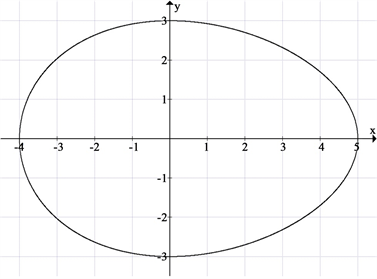Figure 2. Equation (110) graphics

$y=±\frac{3}{6\sqrt{3}}\sqrt{\left(x+3\right){\left(x-6\right)}^{2}},\text{\hspace{0.17em}}\text{\hspace{0.17em}}x\in \left[-3,6\right]$ (111)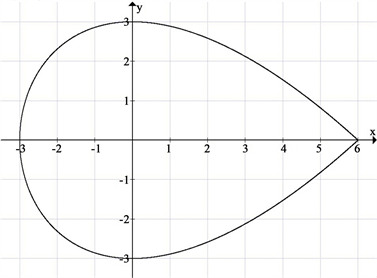Figure 3. Equation (111) graphics

7.2. 仿真验证

$y=±\frac{147}{37080}\sqrt{\left(x+180\right)\left(x-206\right)\left(26x-37080\right)},\text{\hspace{0.17em}}x\in \left[-180,206\right]$ (112)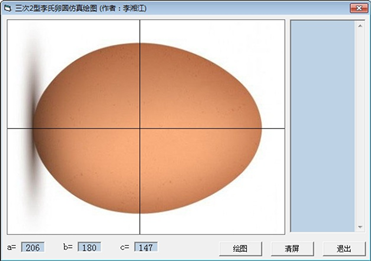(a) 绘图程序界面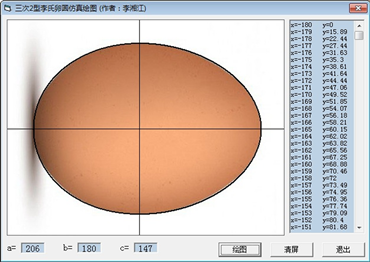(b) 绘图程序运行结果

Figure 4. Drawing program of simulating

8. 结语

  李湘江. 一类四次李氏卵圆[J]. 应用数学进展, 2019, 8(2): 193-202. https://doi.org/10.12677/AAM.2019.82022  李湘江. 一类三次李氏卵圆[J]. 甘肃科学学报, 2020, 32(1): 22-27.  李湘江. 一类参数式李氏卵形曲线[J]. 应用数学进展, 2021, 10(1): 311-327. https://doi.org/10.12677/AAM.2021.101036  同济大学应用数学系. 高等数学第五版上册[M]. 北京: 高等教育出版社, 2002: 148, 153, 269, 273, 278.  同济大学应用数学系. 高等数学第五版下册[M]. 北京: 高等教育出版社, 2002: 145.  Г.М.菲赫金哥尔茨. 微积分学教程二册一分册[M]. 北京大学高等数学教研组, 译. 北京: 人民教育出版社, 1959: 21.